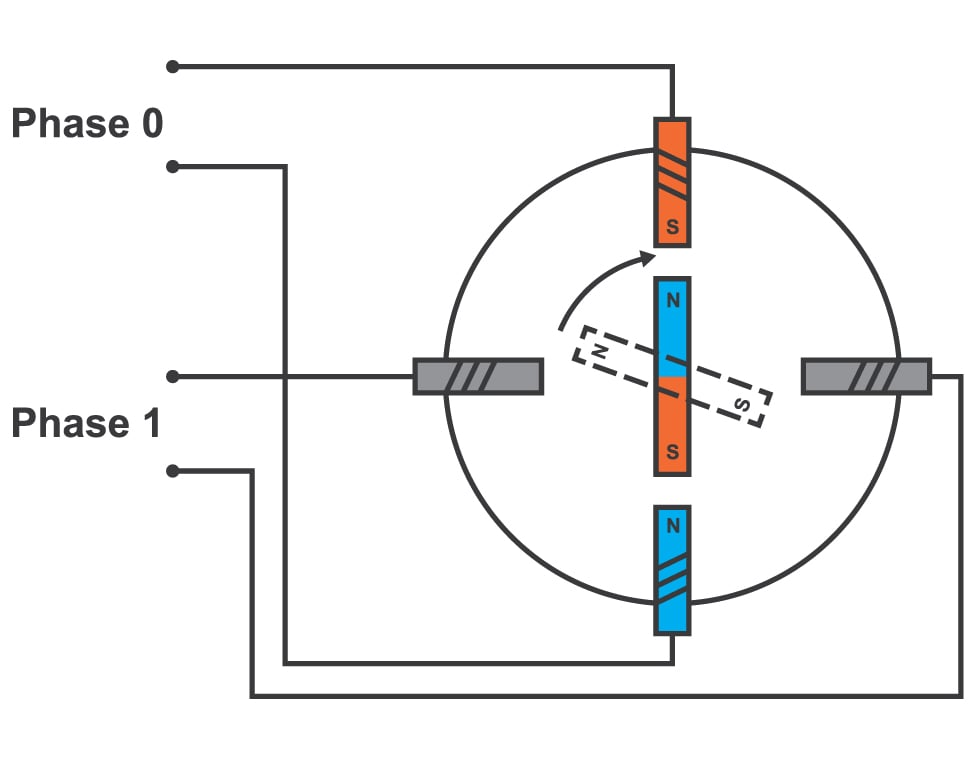# Stepper Motor Calculator

## A tool designed to calculate the maximum speed, minimum time per step and maximum power of a stepper motor

Amps (A)
milliHenry (mH)
Volts (V)
Steps

revolutions/sec
ms
Watts

### OverviewA stepper motor is a popular type of synchronous motor because it allows for precise movements or "steps". This type of motor also produces a lot of torque given it size which is why it has found itself in a number of industrial applications.

This tool is designed to calculate the maximum speed of a stepper motor as well as the minimum time per step and the maximum power dissipation. To use this calculator, simply supply the required fields and click the "calculate" button.

### Equations

$$\text{Max Speed} = \frac{V}{2LI_{max}\cdot spr}$$

$$\text{Minimum Time per Step} = \frac{2LI_{max}}{V}$$

$$P_{max} = I_{max}V$$

Where:

$$V$$ = applied voltage

$$I_{max}$$ = maximum current

$$L$$ = stepper motor inductance

$$spr$$ = steps per revolution

### Applications

Stepper motors are often computer-controlled and can be categorized under motion-control positioning. In this application, the stepper motor is part of an open loop system for holding or positioning objects.

Because they allow very small movements, stepper motors are also found in the field of lasers and optics where precision positioning is often required. These motors are often part of linear actuators, goniometers and rotation stages. Similarly, stepper motors are used in fluid control systems particularly in positioning valve pilot stages. They can also be found in packaging machinery.

The current 3D printers and CNC machines also use stepper motors as actuators. Prior to these technologies, the stepper motor has been used in disk drives, printers, slot machines, camera lenses and image scanners.•Michael Shievitz March 19, 2017

Could you clarify how microstepping drivers affect your calculator, is it simply input the number of desired microsteps, or the physical stepping of the motor?

eg 1.8 degree motors have 200 full steps per revolution, 0.9 400 full steps per revolution.

Like.
•David_Francis September 17, 2022
Taking as example the 1.8 degree motor, the minimum number of steps per revolution is 200, but a microstepping driver can apply varying ratios of current to the A & B windings to create intermediate steps.
Like.
• T
tomhab May 02, 2017